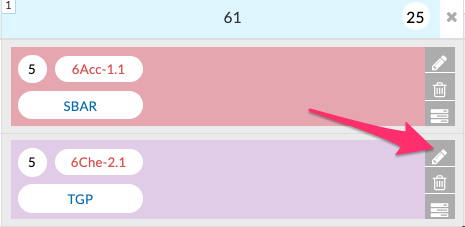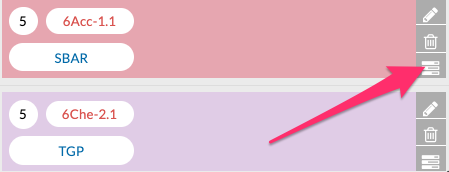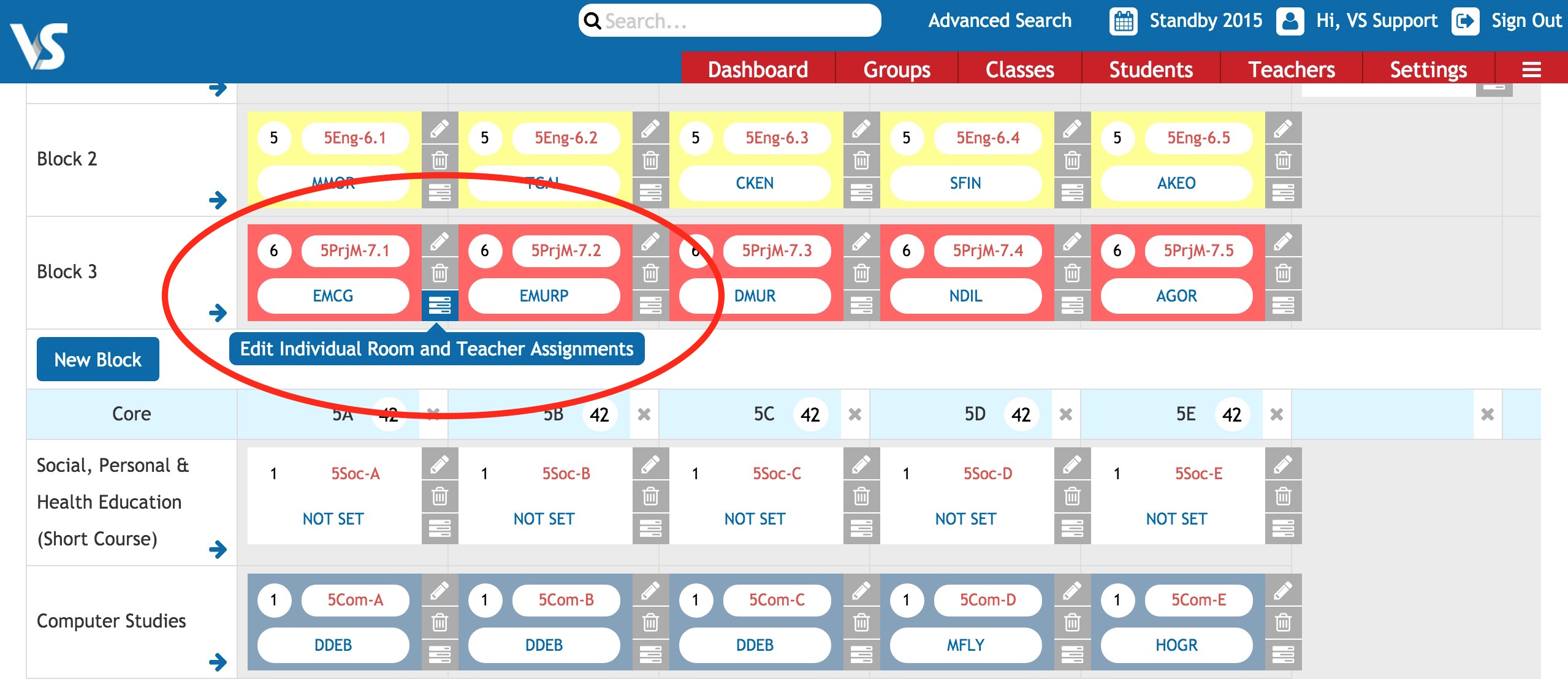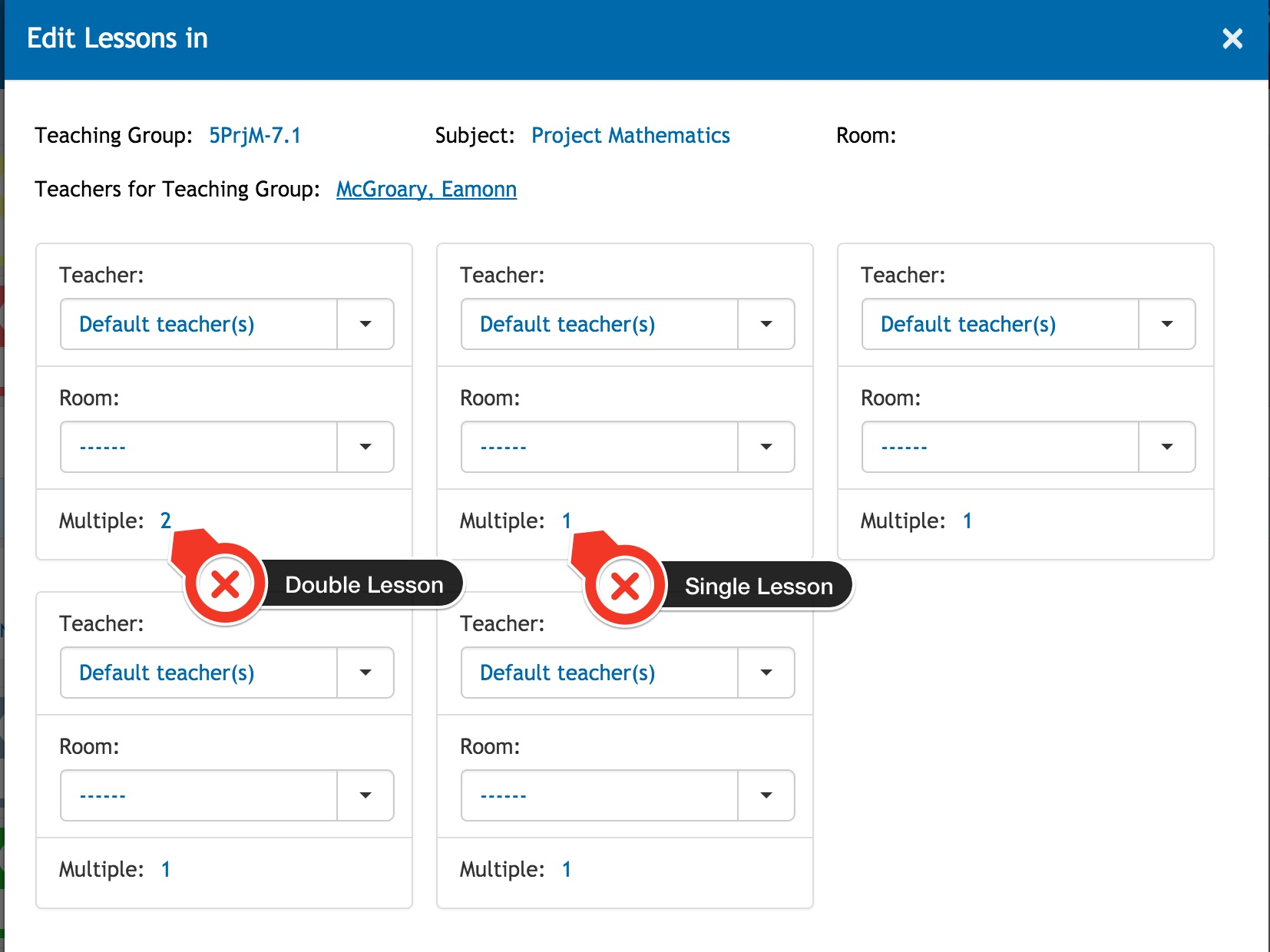Help Center
1. Help Center
2. Timetabling
3. General Timetabling

# Lesson Spread in Timetable Construction (Step 5) - Class Data

Last updated 18/07/2022

Spread is the term we use to describe the precise breakdown for the lessons of a class on the timetable grid. The 'spread' tells our solver how you wish the lessons to be placed on the grid.

### Step 1

To specify how lessons are to be scheduled, first go to Timetable Construction (Step 5) - Class Data > click on the edit pencil beside a teaching group.• (1,1,1,1,1 means five lessons should be single lessons spread over five days)
• (2,1,1,1 means the five lessons should have one double lesson and three single lessons)
• (2,2,1 means you require two double lessons and one single class)
• (3,2 means a treble lesson and a double lesson)
• (Blank means that you do not mind what the spread will be. The numbers in the spread cannot be greater than the number of lessons in total)

### Step 2

You can also click on the 3 lines icon for Individual Lessons, you will see the lessons displayed according to the spread entry.Please examine the examples below to see the syntax we use for 'spread' in VSware's Timetable Construction:

• 5 lessons of English could be scheduled with a spread of 1,1,1,1,1. This means that we want five single lessons and the solver will endeavour to schedule these lesson over all five days of the school week. The number represents the amount of lessons in a day, and each comma represents a separate day. This spread might be ideal for lessons in English but might not be suitable if these were lessons in a practical subject.
• 5 lessons of Biology could be scheduled with a spread of 2,1,1,1. In this case the solver will try to schedule 1 double lesson and 3 single lessons over the course of the week. This is usually suitable for subjects with a practical element. It also means that there will be 1 day during the week when the lesson will not take place. Another spread that could be used for subjects with a practical element is 2,2,1. This would schedule 2 double lessons and 1 single lesson.
• A PE class with 2 lesson will usually have a spread of 2. This will schedule a double lesson.
• 10 lessons of a class with a spread of 3,3,2,1,1 would try to schedule 2 treble lessons, 1 double lesson and 2 single lessons. (In the Further Education sector, spreads like this are often requested).

6 or more lessons can be spread over five days but we must enter how we want this to occur. When there is more than 5 lessons the solver will need to schedule more than one lesson on at least one day. You must tell the solver exactly how you would like this to occur.

Here are two examples of 6 lessons of Maths spread over the week

• 6 lessons with a spread of 2,1,1,1,1 will schedule a double lesson and 4 single lessons over the five days of the week.
• 6 lessons with a spread of -2,1,1,1,1 will schedule 2 single lessons on one day and 4 single lessons for the remaining days. The '-2' in the spread tells the solver to schedule 2 lessons on the same day but these must not be a double lesson.

***Please Note: The spread of each class in a normal block must be identical.

If you do not enter any spread or the spread entered is less than the number of lessons, the solver will schedule these where is can find a free period slot. This is a lot less restrictive and can be useful in cases where it does not really matter if single or double lessons are scheduled.

If you still have questions, please visit our knowledge base at support.vsware.ie. You can contact our support team via email at support@vsware.ie, via the chat bubble when you are logged into your VSware account or via phone on 01-5549628. Support hours are Mon - Fri, 08:30 - 18:00.